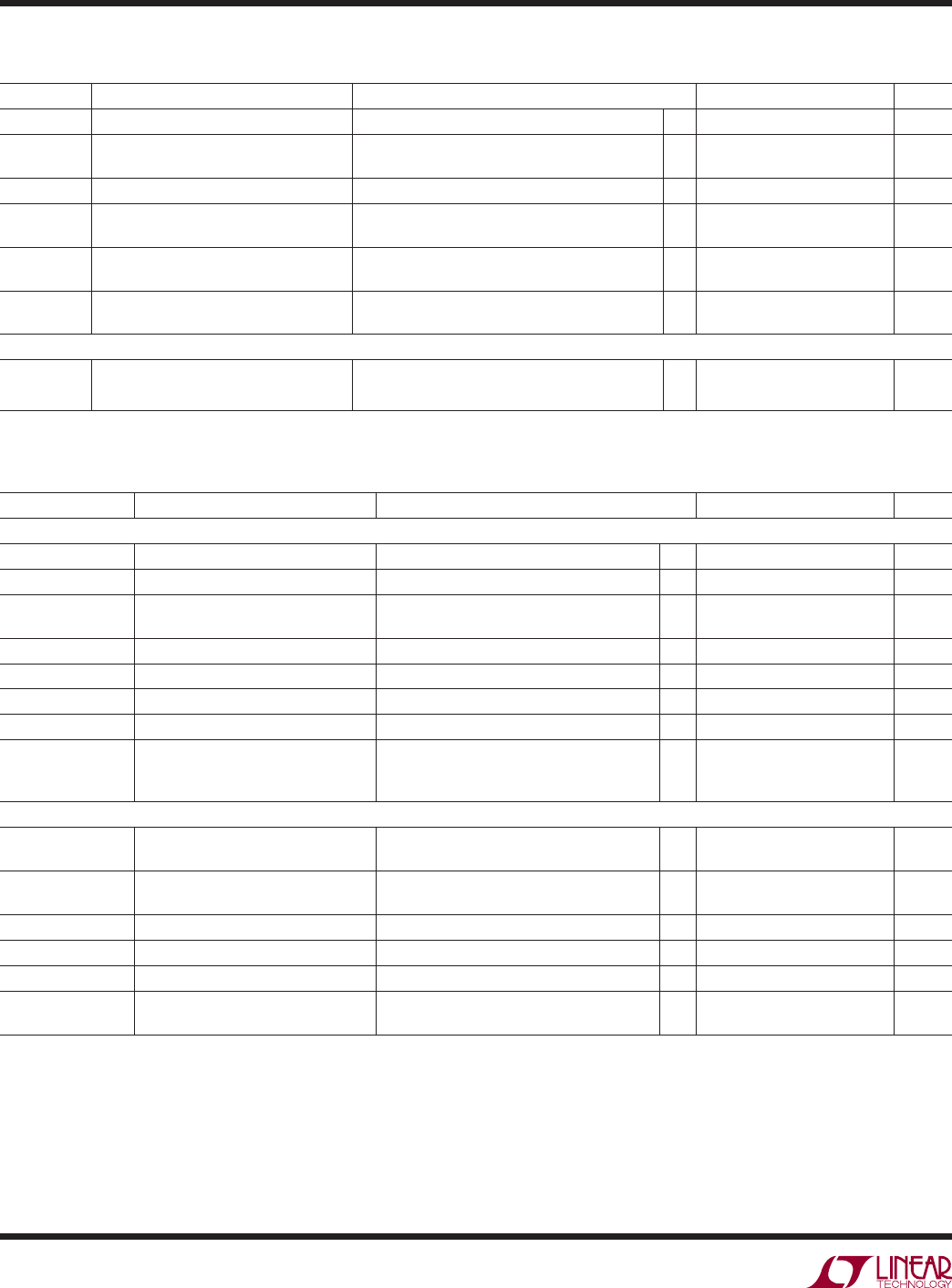# DatasheetLTC2854/LTC2855
4
285455fb
SYMBOL PARAMETER CONDITIONS MIN TYP MAX UNITS
Driver
f
MAX
Maximum Data Rate Note 3
20 Mbps
t
PLHD
, t
PHLD
Driver Input to Output R
DIFF
= 54Ω, C
L
= 100pF (Figure 4)
10 50 ns
Δt
PD
Driver Input to Output Difference
|t
PLHD
-t
PHLD
|
R
DIFF
= 54Ω, C
L
= 100pF (Figure 4)
16 ns
t
SKEWD
Driver Output Y to Output Z R
DIFF
= 54Ω, C
L
= 100pF (Figure 4)
6 ns
t
RD
, t
FD
Driver Rise or Fall Time R
DIFF
= 54Ω, C
L
= 100pF (Figure 4)
412.5 ns
t
ZLD
, t
ZHD
, t
LZD
, t
HZD
Driver Enable or Disable Time R
L
= 500Ω, C
L
= 50pF, RE = 0 (Figure 5)
70 ns
t
ZHSD
, t
ZLSD
Driver Enable from Shutdown R
L
= 500Ω, C
L
= 50pF, RE = V
CC
(Figure 5)
s
t
SHDN
Time to Shutdown R
L
= 500Ω, C
L
= 50pF
(DE = , RE = V
CC
) or (DE = 0, RE )
(Figure 5)
100 ns
t
PLHR
, t
PHLR
L
= 15pF, V
CM
= 1.5V, |V
AB
| = 1.5V, t
R
and t
F
< 4ns (Figure 6)
50 70 ns
t
SKEWR
|t
PLHR
-t
PHLR
|
C
L
= 15pF (Figure 6)
16 ns
t
RR
, t
FR
Receiver Output Rise or Fall Time C
L
= 15pF (Figure 6)
3 12.5 ns
t
ZLR
, t
ZHR
, t
LZR
, t
HZR
L
= 1k, C
L
=15pF, DE = V
CC
(Figure 7)
50 ns
t
ZHSR
, t
ZLSR
L
= 1k, C
L
= 15pF, DE = 0V (Figure 7)
s
t
RTEN
, t
RTZ
Termination Enable or Disable Time V
B
= 0V, V
AB
= 2V, RE = V
CC
, DE = 0V
(Figure 8)
100 μs
Note 1: Stresses beyond those listed under Absolute Maximum Ratings
may cause permanent damage to the device. Exposure to any Absolute
Maximum Rating condition for extended periods may affect device
Operating lifetime is derated at temperatures greater than 105°C
Note 2: All currents into device pins are positive; all currents out of device
pins are negative. All voltages are referenced to device ground unless
otherwise speciﬁ ed.
SYMBOL PARAMETER CONDITIONS MIN TYP MAX UNITS
I
CCT
Supply Current in Transmit Mode No Load, DE = V
CC
, RE = V
CC
, TE = 0V
450 1000 μA
I
CCTR
Supply Current with Both Driver and
CC
, RE = 0V, TE = 0V
450 1000 μA
I
CCTERM
Supply Current in Termination Mode DE = 0V, RE = V
CC
, TE = V
CC
110 180 μA
I
CCTERMR
Termination Mode
DE = 0V, RE = 0V, TE = V
CC
450 950 μA
I
CCTERMT
Supply Current in Transmit and
Termination Mode
DE = V
CC
, RE = V
CC
, TE = V
CC
470 1000 μA
I
CCTERMTR
and Termination Enabled
DE = V
CC
, RE = 0V, TE = V
CC
470 1000 μA
ESD Protection
ESD Protection for RS485/RS422 Pins A, B on LTC2854, Human Body Model
Y, Z, A, B on LTC2855, Human Body Model
±25
±15
kV
kV
The denotes the speciﬁ cations which apply over the full operating
temperature range, otherwise speciﬁ cations are at T
A
= 25°C, V
CC
= 3.3V, T
E
= 0V unless otherwise noted (Note 2).
Note 3: Maximum data rate is guaranteed by other measured parameters
and is not tested directly.
Note 4: This IC includes overtemperature protection that is intended
to protect the device during momentary overload conditions.
Overtemperature protection activates at a junction temperature exceeding
150°C. Continuous operation above the speciﬁ ed maximum operating
junction temperature may result in device degradation or failure.
The denotes the speciﬁ cations which apply over the full operating
temperature range, otherwise speciﬁ cations are at T
A
= 25°C, V
CC
= 3.3V, T
E
= 0V unless otherwise noted (Note 2).
ELECTRICAL CHARACTERISTICS
SWITCHING CHARACTERISTICS